Скачать презентацию Let s Look at Math Some Equations and Applications

23f772de229faf45da639654387a4117.ppt

• Количество слайдов: 50Let’s Look at Math Some Equations and Applications for Drinking Water Operations Patricia Quinn and Russell KoenigPatricia Quinn - Environmental Health and Water Quality Technology Student at Milwaukee Area Technical College, Certified Surface Water Treatment Operator, WAV Monitor, Member, WIAWWA Russell Koenig - Environmental Health and Water Quality Technology Student at Milwaukee Area Technical College, Certified Surface Water Treatment Operator, interned at Northshore Water Commission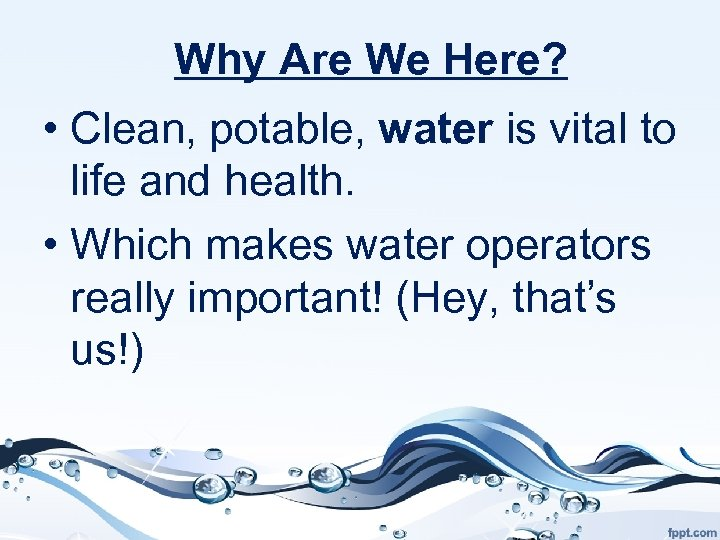Why Are We Here? • Clean, potable, water is vital to life and health. • Which makes water operators really important! (Hey, that’s us!)Why Are We Here? • Way more important in case of a zombie apocalypse…sorry doctors.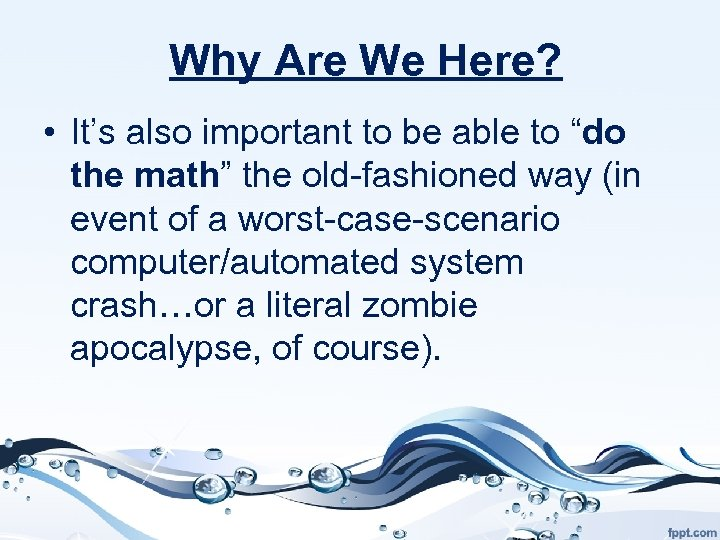Why Are We Here? • It’s also important to be able to “do the math” the old-fashioned way (in event of a worst-case-scenario computer/automated system crash…or a literal zombie apocalypse, of course).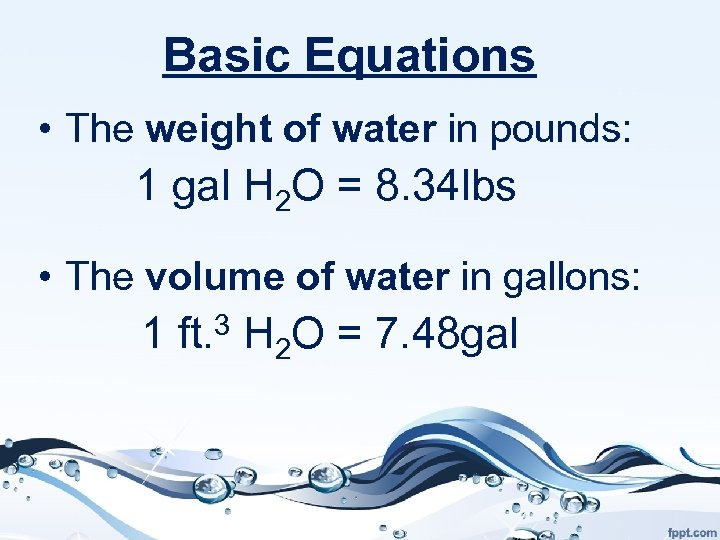Basic Equations • The weight of water in pounds: 1 gal H 2 O = 8. 34 lbs • The volume of water in gallons: 1 ft. 3 H 2 O = 7. 48 gal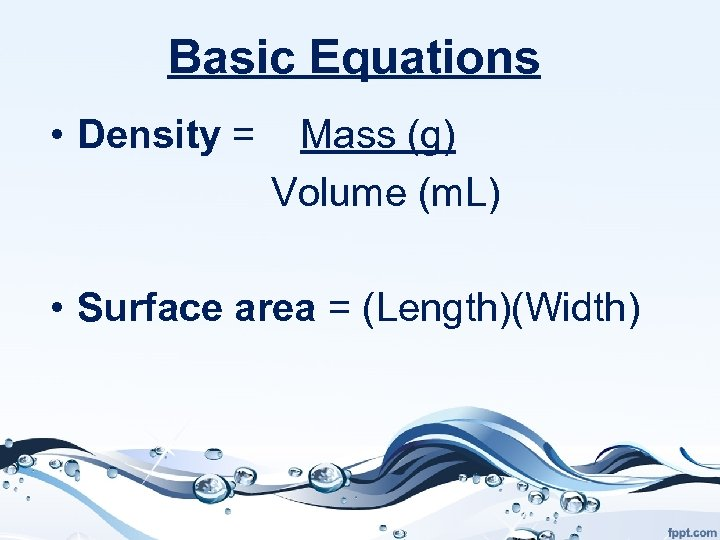Basic Equations • Density = Mass (g) Volume (m. L) • Surface area = (Length)(Width)Basic Equations • Velocity: V = Distance Time • Flow: Q = AV (Area)(Velocity)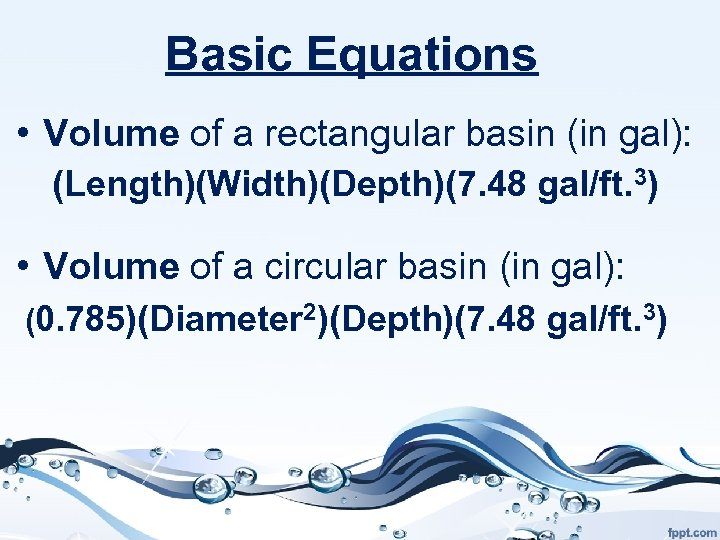Basic Equations • Volume of a rectangular basin (in gal): (Length)(Width)(Depth)(7. 48 gal/ft. 3) • Volume of a circular basin (in gal): (0. 785)(Diameter 2)(Depth)(7. 48 gal/ft. 3)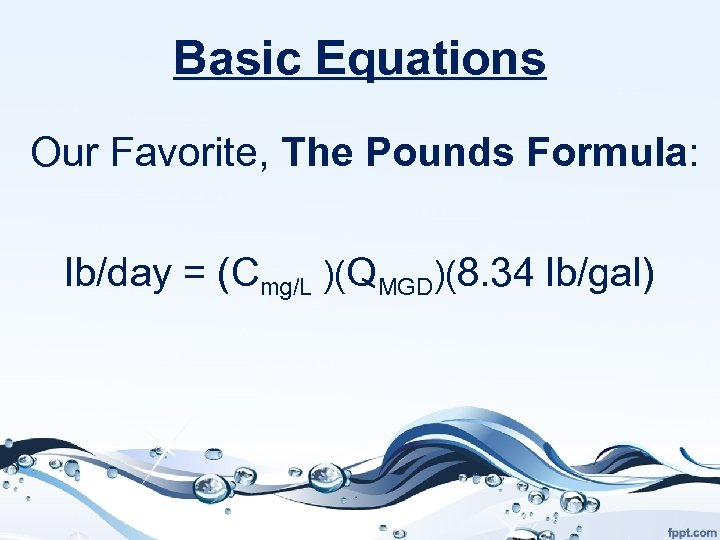Basic Equations Our Favorite, The Pounds Formula: lb/day = (Cmg/L )(QMGD)(8. 34 lb/gal)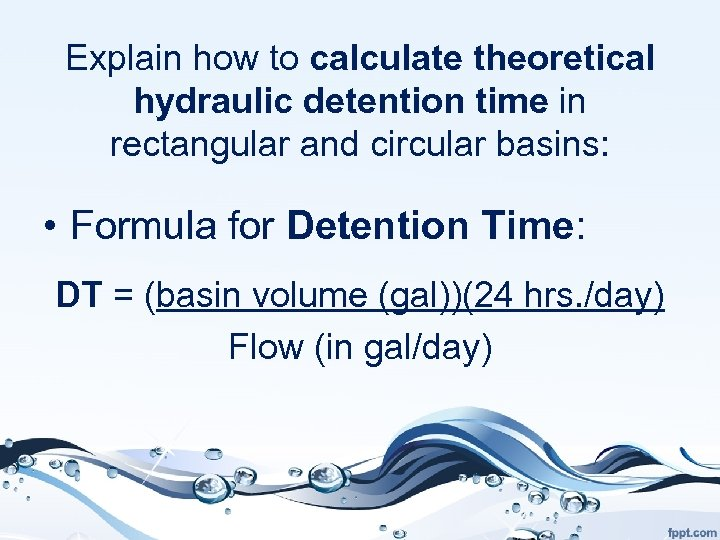Explain how to calculate theoretical hydraulic detention time in rectangular and circular basins: • Formula for Detention Time: DT = (basin volume (gal))(24 hrs. /day) Flow (in gal/day)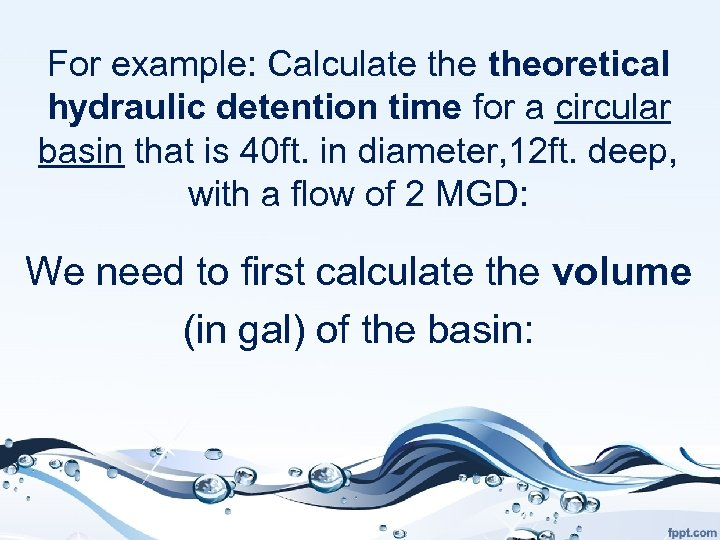For example: Calculate theoretical hydraulic detention time for a circular basin that is 40 ft. in diameter, 12 ft. deep, with a flow of 2 MGD: We need to first calculate the volume (in gal) of the basin: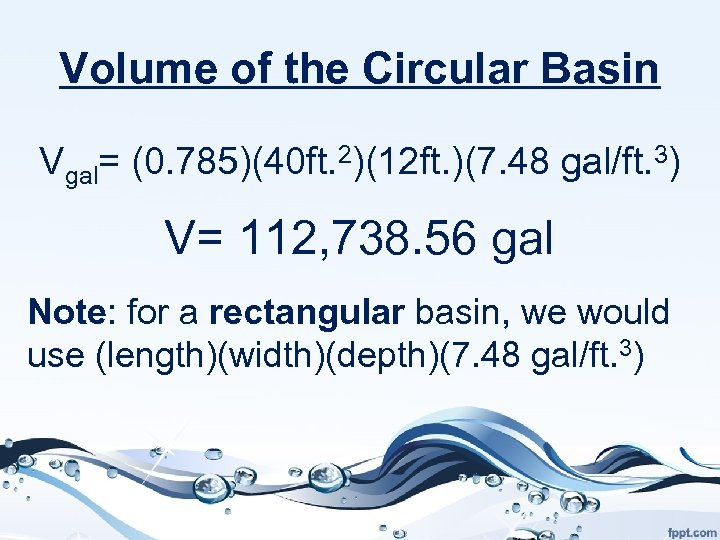Volume of the Circular Basin Vgal= (0. 785)(40 ft. 2)(12 ft. )(7. 48 gal/ft. 3) V= 112, 738. 56 gal Note: for a rectangular basin, we would use (length)(width)(depth)(7. 48 gal/ft. 3)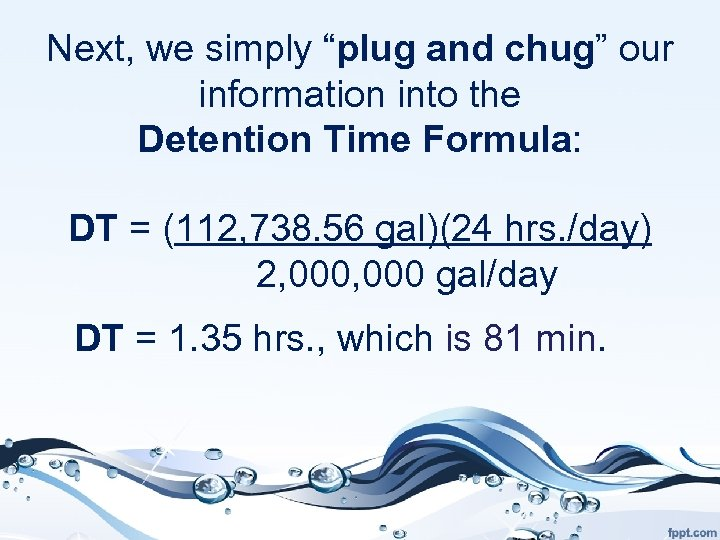Next, we simply “plug and chug” our information into the Detention Time Formula: DT = (112, 738. 56 gal)(24 hrs. /day) 2, 000 gal/day DT = 1. 35 hrs. , which is 81 min.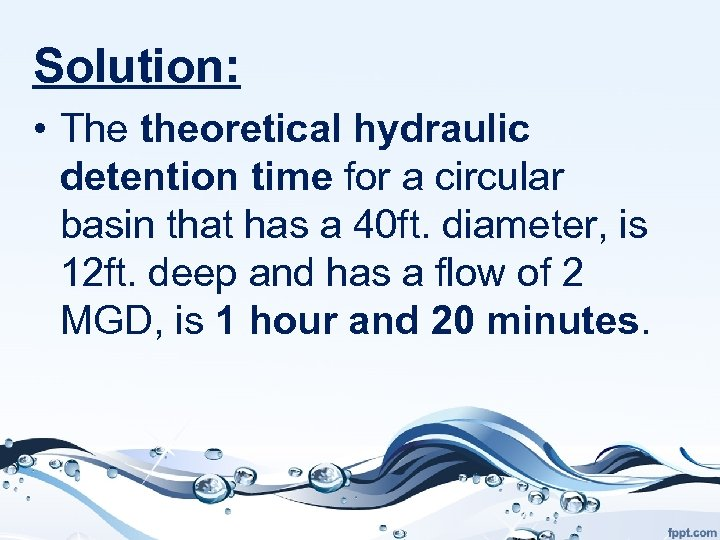Solution: • The theoretical hydraulic detention time for a circular basin that has a 40 ft. diameter, is 12 ft. deep and has a flow of 2 MGD, is 1 hour and 20 minutes.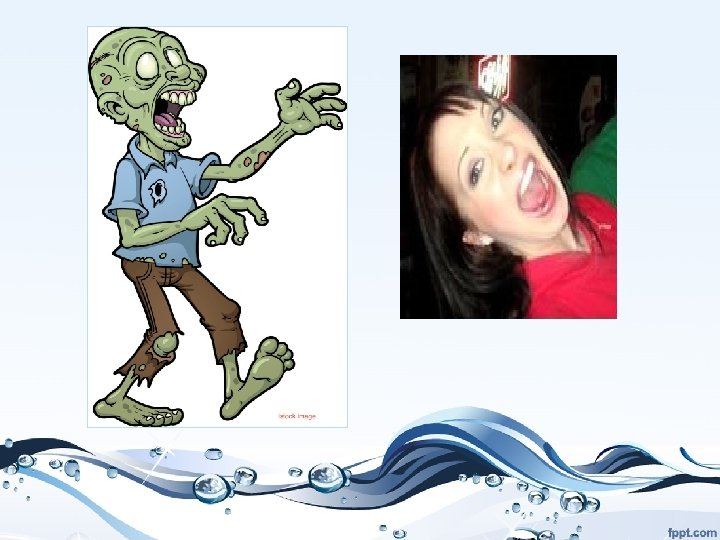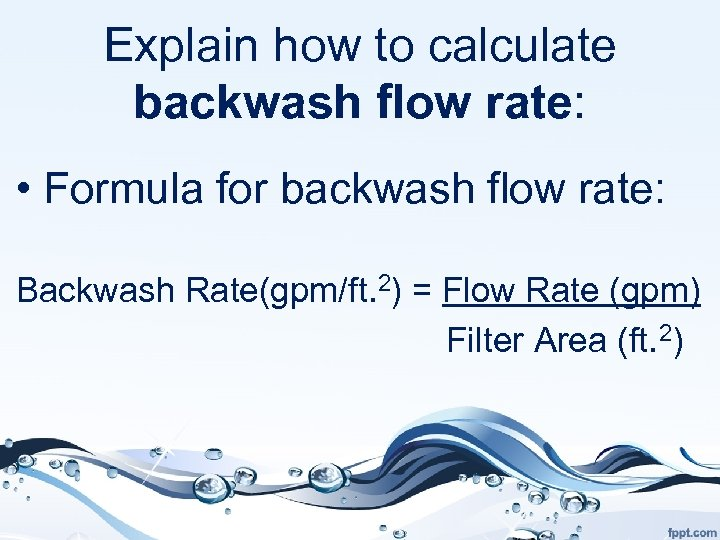Explain how to calculate backwash flow rate: • Formula for backwash flow rate: Backwash Rate(gpm/ft. 2) = Flow Rate (gpm) Filter Area (ft. 2)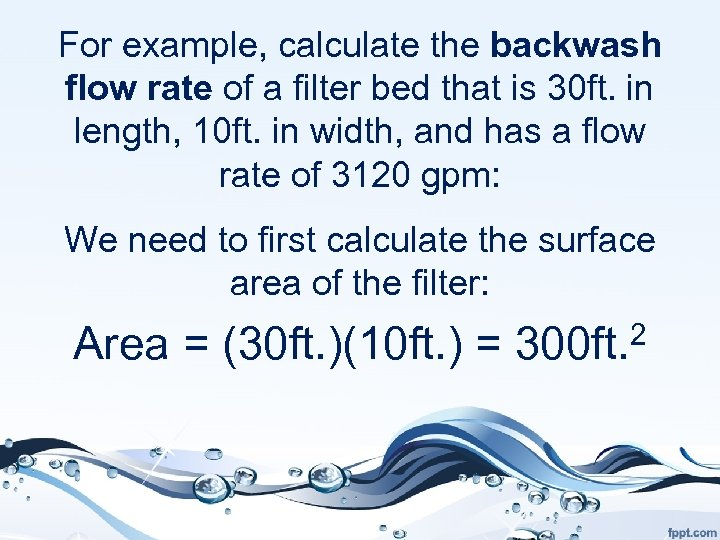For example, calculate the backwash flow rate of a filter bed that is 30 ft. in length, 10 ft. in width, and has a flow rate of 3120 gpm: We need to first calculate the surface area of the filter: Area = (30 ft. )(10 ft. ) = 300 ft. 2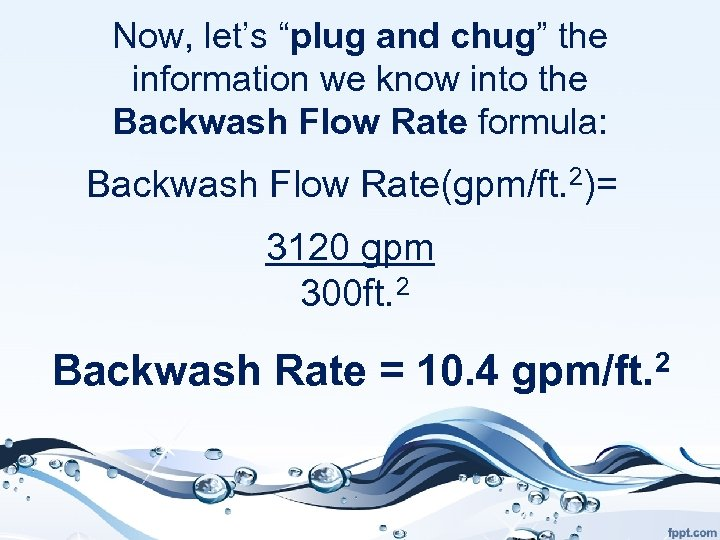Now, let’s “plug and chug” the information we know into the Backwash Flow Rate formula: Backwash Flow Rate(gpm/ft. 2)= 3120 gpm 300 ft. 2 Backwash Rate = 10. 4 gpm/ft. 2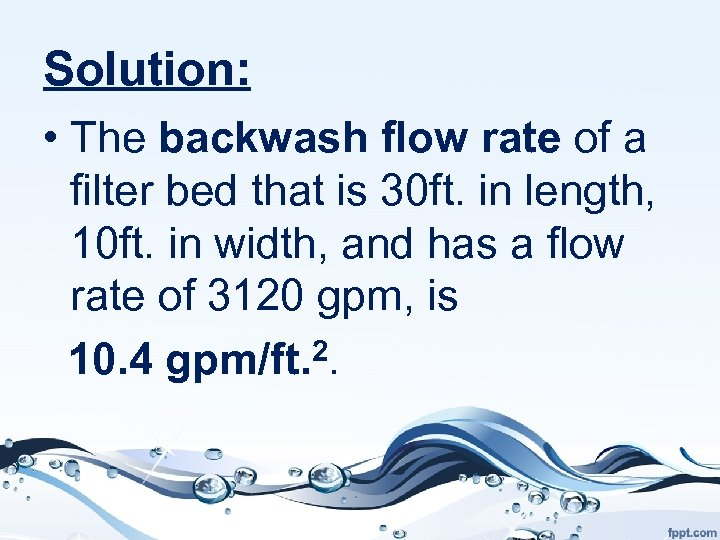Solution: • The backwash flow rate of a filter bed that is 30 ft. in length, 10 ft. in width, and has a flow rate of 3120 gpm, is 10. 4 gpm/ft. 2.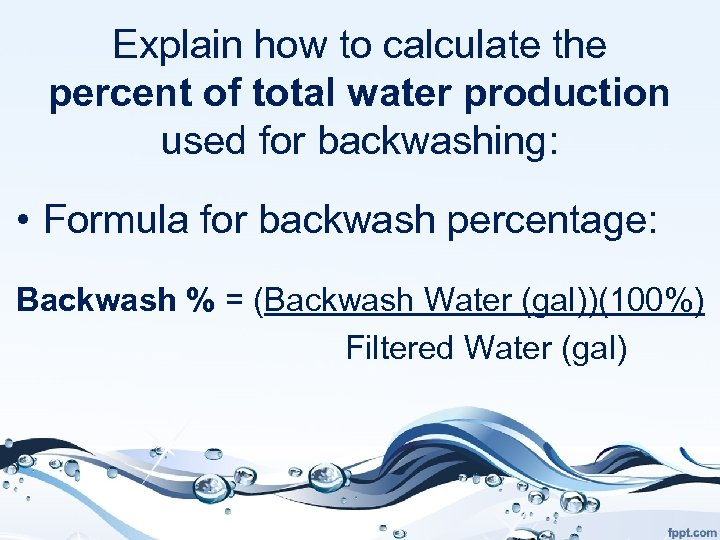Explain how to calculate the percent of total water production used for backwashing: • Formula for backwash percentage: Backwash % = (Backwash Water (gal))(100%) Filtered Water (gal)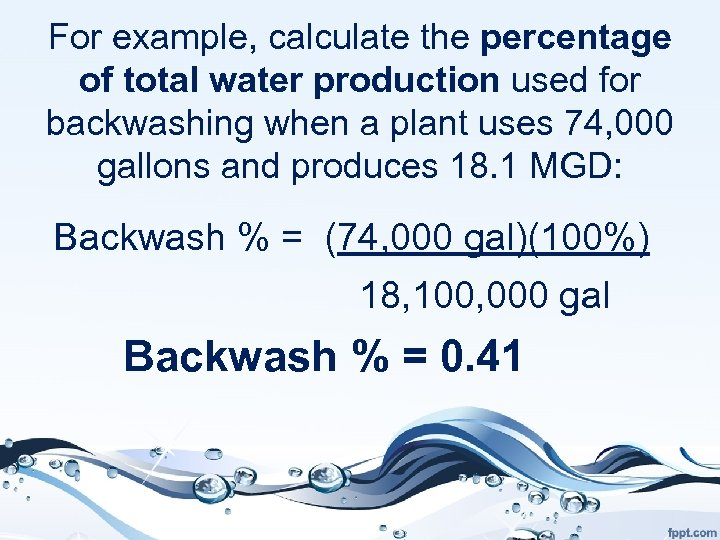For example, calculate the percentage of total water production used for backwashing when a plant uses 74, 000 gallons and produces 18. 1 MGD: Backwash % = (74, 000 gal)(100%) 18, 100, 000 gal Backwash % = 0. 41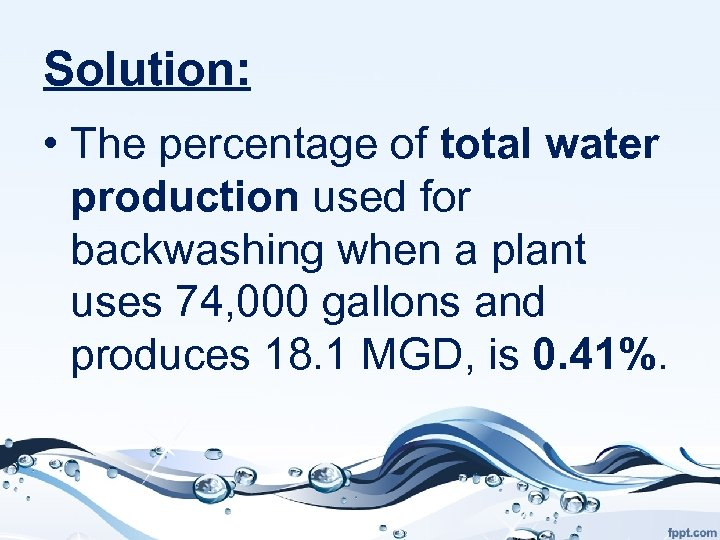Solution: • The percentage of total water production used for backwashing when a plant uses 74, 000 gallons and produces 18. 1 MGD, is 0. 41%.Where do zombies go swimming? THE DEAD SEA!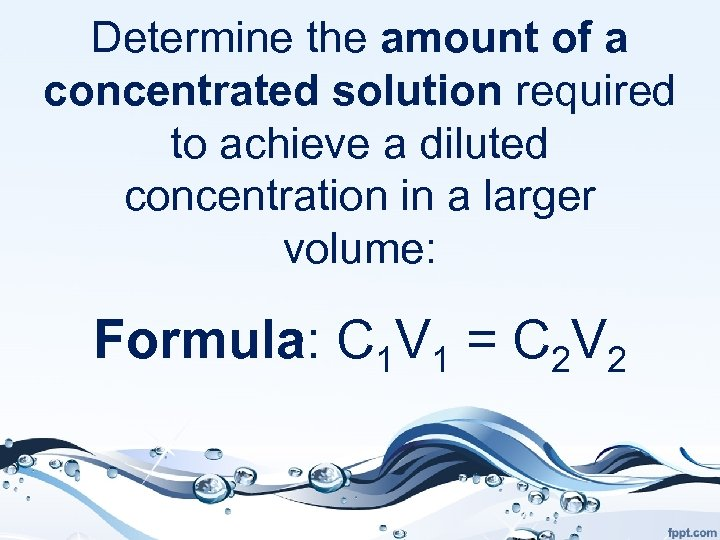Determine the amount of a concentrated solution required to achieve a diluted concentration in a larger volume: Formula: C 1 V 1 = C 2 V 2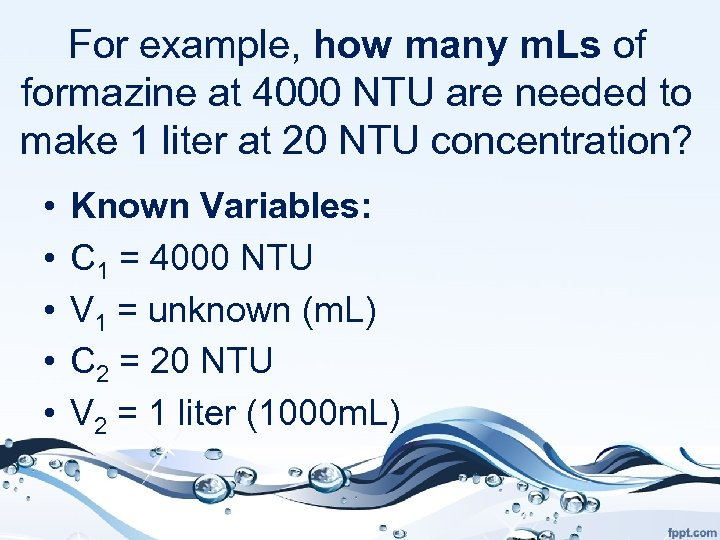For example, how many m. Ls of formazine at 4000 NTU are needed to make 1 liter at 20 NTU concentration? • • • Known Variables: C 1 = 4000 NTU V 1 = unknown (m. L) C 2 = 20 NTU V 2 = 1 liter (1000 m. L)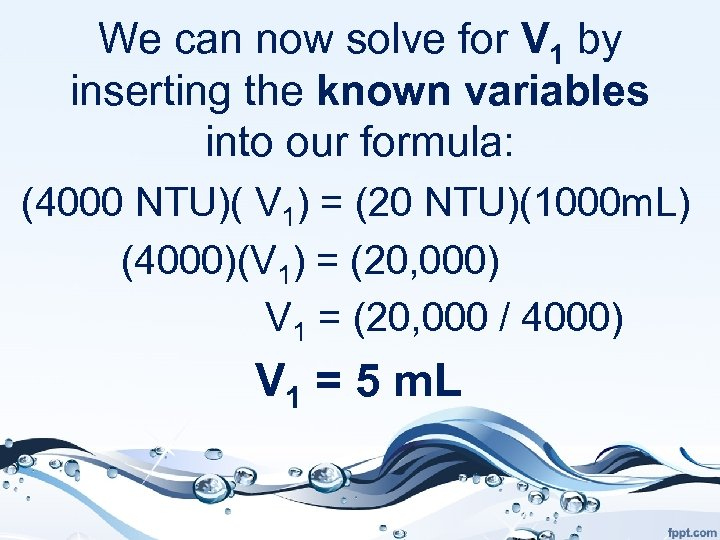We can now solve for V 1 by inserting the known variables into our formula: (4000 NTU)( V 1) = (20 NTU)(1000 m. L) (4000)(V 1) = (20, 000) V 1 = (20, 000 / 4000) V 1 = 5 m. L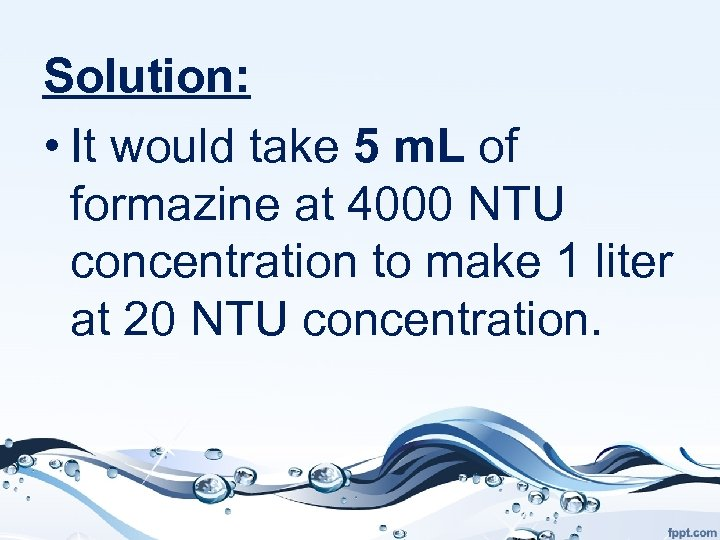Solution: • It would take 5 m. L of formazine at 4000 NTU concentration to make 1 liter at 20 NTU concentration.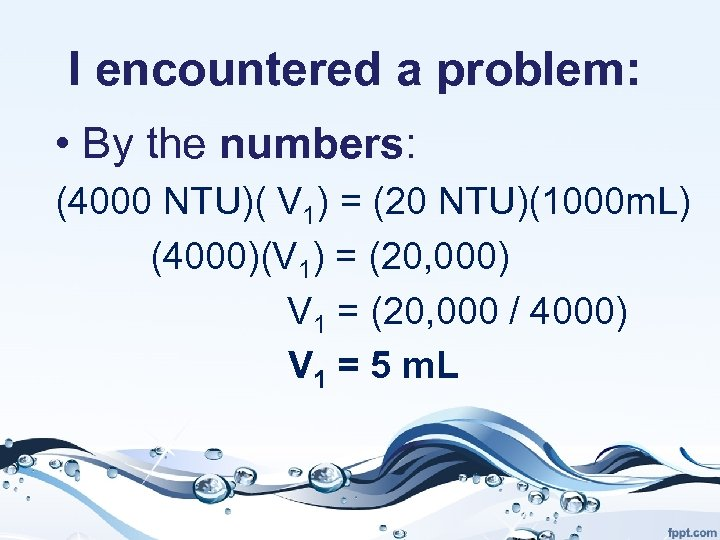I encountered a problem: • By the numbers: (4000 NTU)( V 1) = (20 NTU)(1000 m. L) (4000)(V 1) = (20, 000) V 1 = (20, 000 / 4000) V 1 = 5 m. L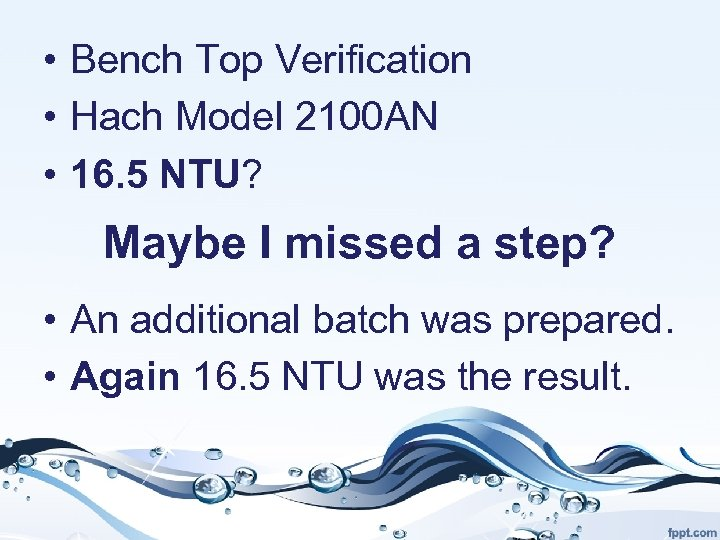• Bench Top Verification • Hach Model 2100 AN • 16. 5 NTU? Maybe I missed a step? • An additional batch was prepared. • Again 16. 5 NTU was the result.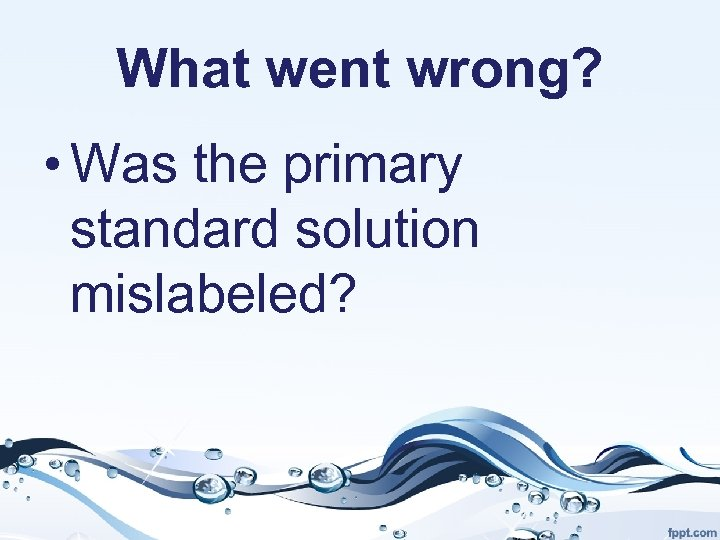What went wrong? • Was the primary standard solution mislabeled?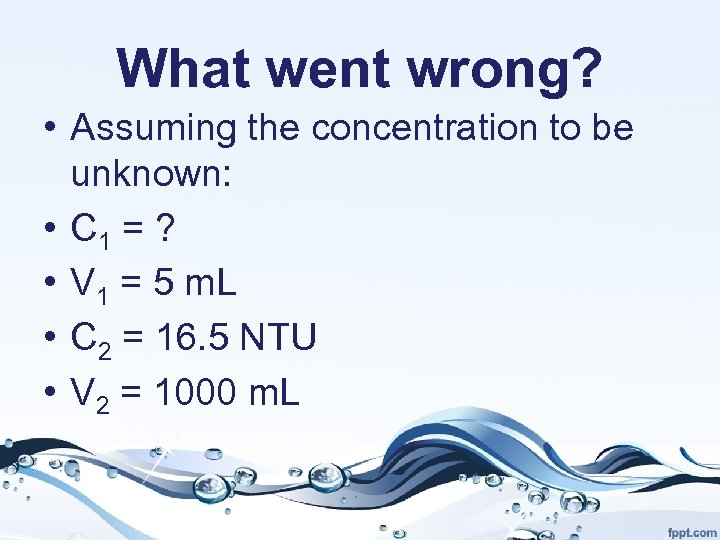What went wrong? • Assuming the concentration to be unknown: • C 1 = ? • V 1 = 5 m. L • C 2 = 16. 5 NTU • V 2 = 1000 m. L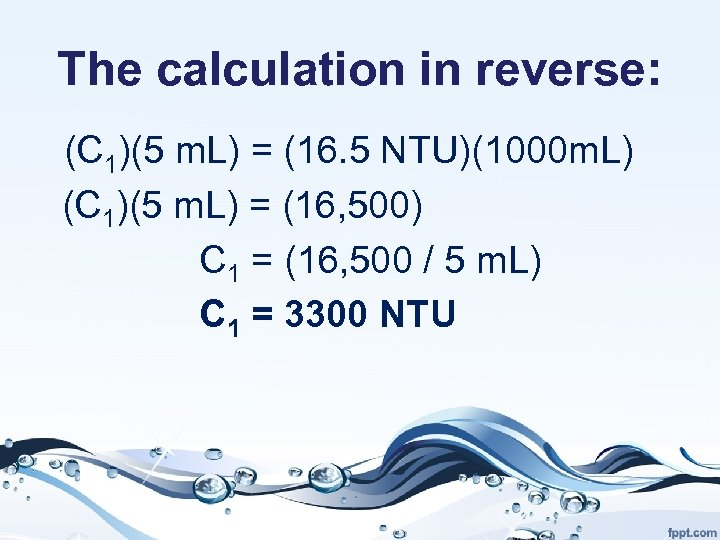The calculation in reverse: (C 1)(5 m. L) = (16. 5 NTU)(1000 m. L) (C 1)(5 m. L) = (16, 500) C 1 = (16, 500 / 5 m. L) C 1 = 3300 NTU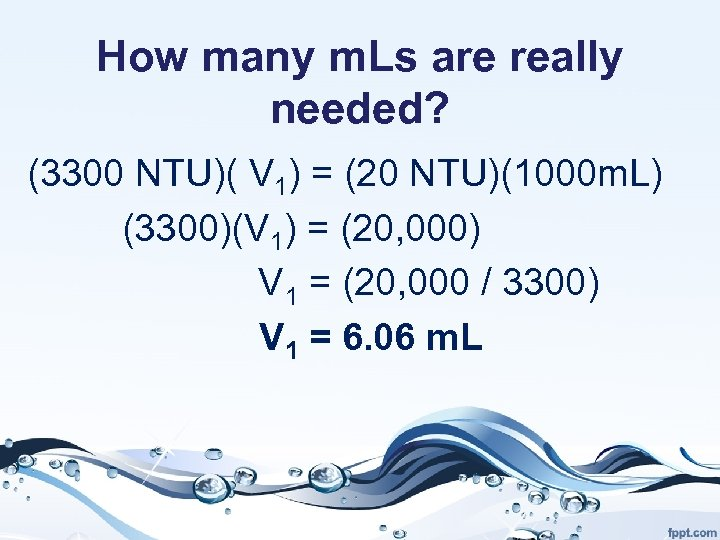How many m. Ls are really needed? (3300 NTU)( V 1) = (20 NTU)(1000 m. L) (3300)(V 1) = (20, 000) V 1 = (20, 000 / 3300) V 1 = 6. 06 m. L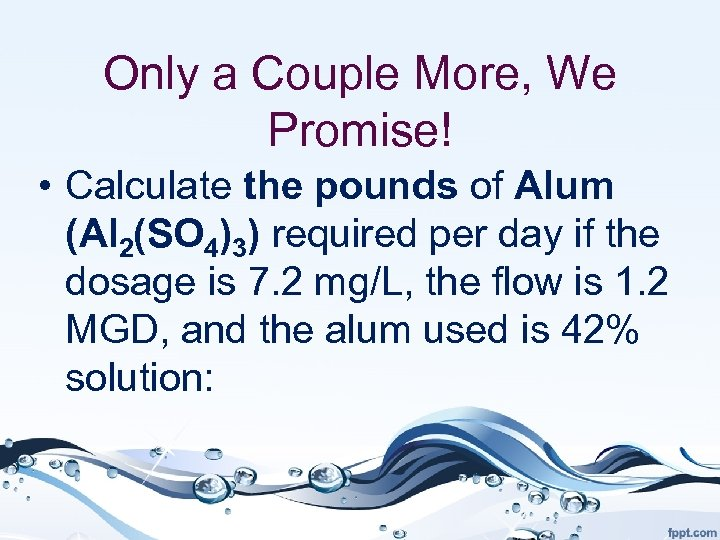Only a Couple More, We Promise! • Calculate the pounds of Alum (Al 2(SO 4)3) required per day if the dosage is 7. 2 mg/L, the flow is 1. 2 MGD, and the alum used is 42% solution: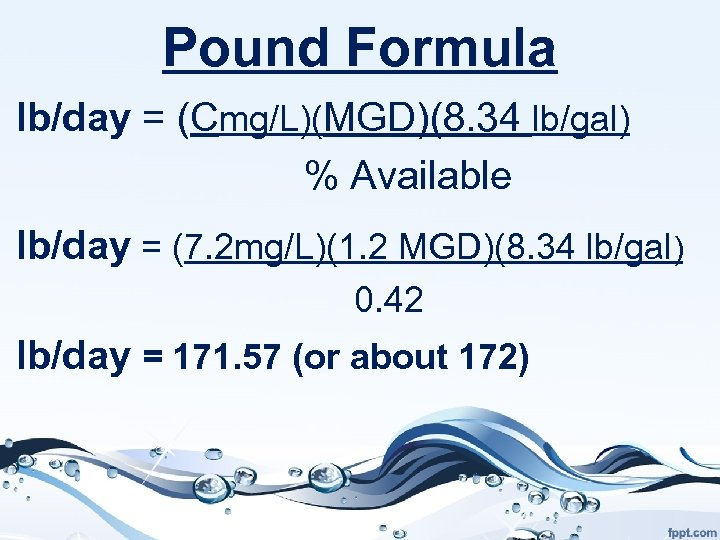Pound Formula lb/day = (Cmg/L)(MGD)(8. 34 lb/gal) % Available lb/day = (7. 2 mg/L)(1. 2 MGD)(8. 34 lb/gal) 0. 42 lb/day = 171. 57 (or about 172)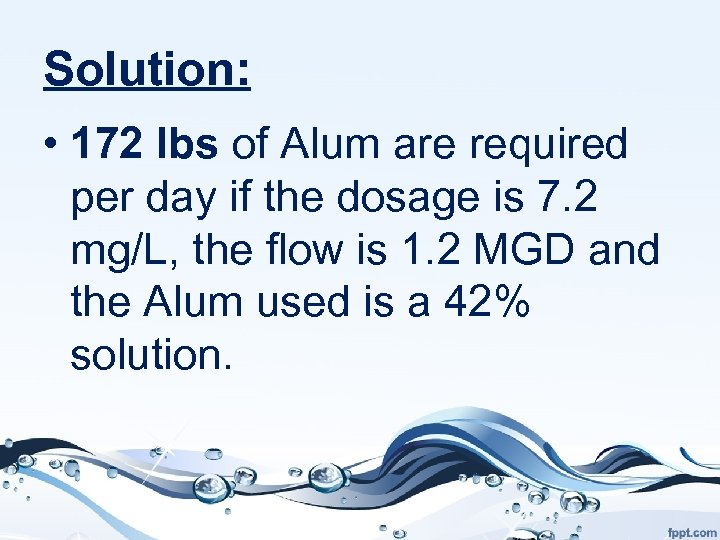Solution: • 172 lbs of Alum are required per day if the dosage is 7. 2 mg/L, the flow is 1. 2 MGD and the Alum used is a 42% solution.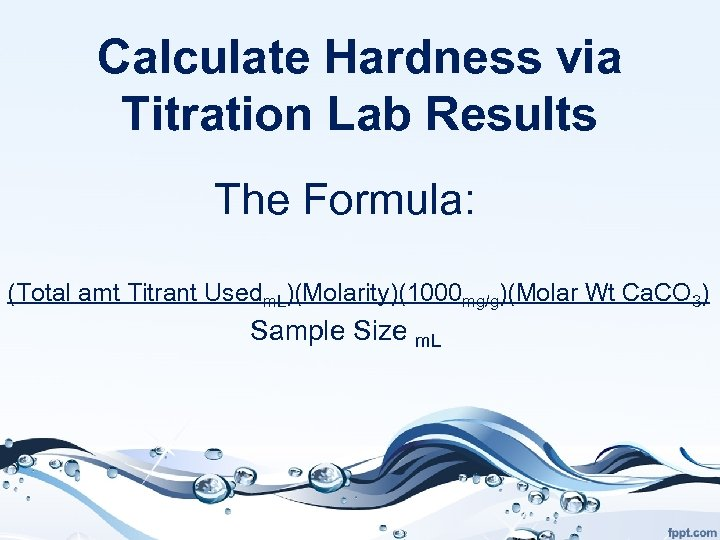Calculate Hardness via Titration Lab Results The Formula: (Total amt Titrant Usedm. L)(Molarity)(1000 mg/g)(Molar Wt Ca. CO 3) Sample Size m. L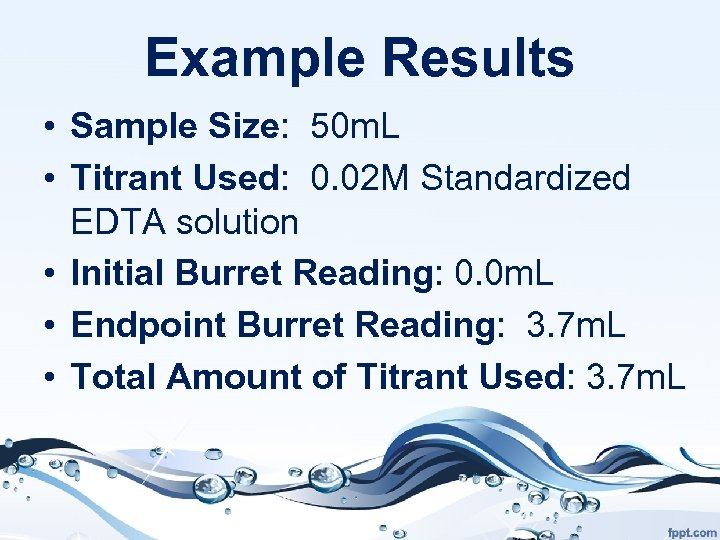Example Results • Sample Size: 50 m. L • Titrant Used: 0. 02 M Standardized EDTA solution • Initial Burret Reading: 0. 0 m. L • Endpoint Burret Reading: 3. 7 m. L • Total Amount of Titrant Used: 3. 7 m. L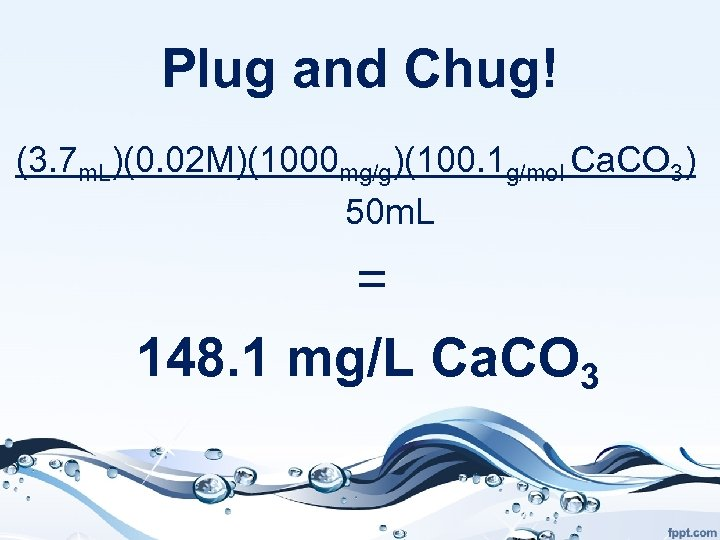Plug and Chug! (3. 7 m. L)(0. 02 M)(1000 mg/g)(100. 1 g/mol Ca. CO 3) 50 m. L = 148. 1 mg/L Ca. CO 3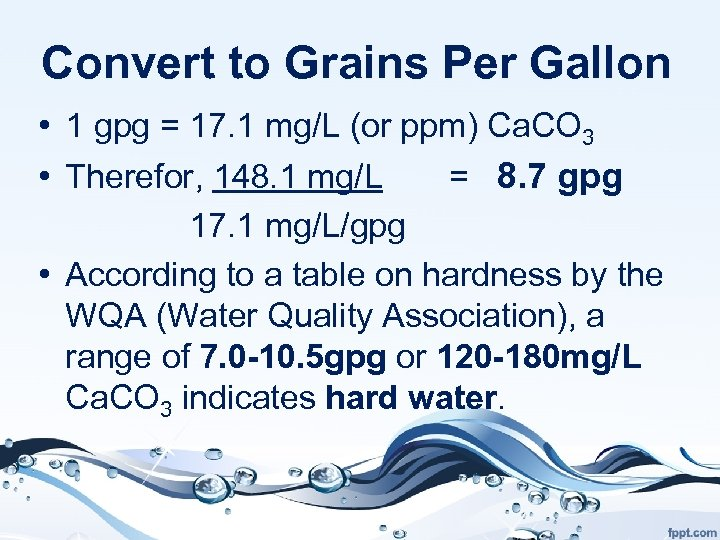Convert to Grains Per Gallon • 1 gpg = 17. 1 mg/L (or ppm) Ca. CO 3 • Therefor, 148. 1 mg/L = 8. 7 gpg 17. 1 mg/L/gpg • According to a table on hardness by the WQA (Water Quality Association), a range of 7. 0 -10. 5 gpg or 120 -180 mg/L Ca. CO 3 indicates hard water.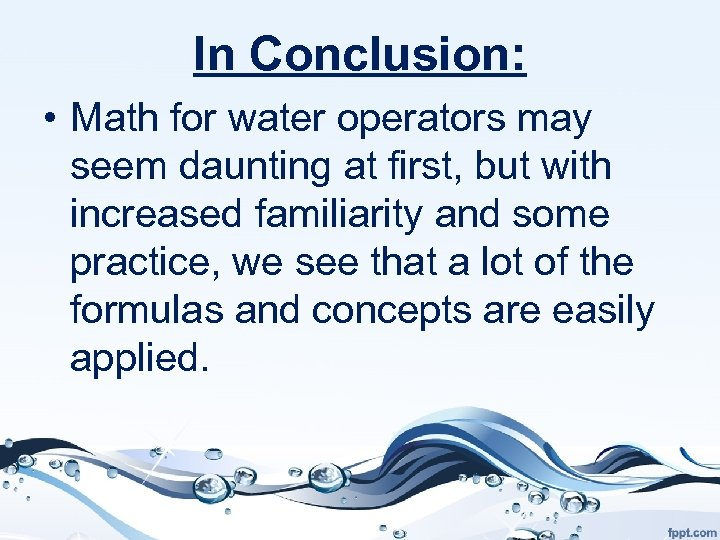In Conclusion: • Math for water operators may seem daunting at first, but with increased familiarity and some practice, we see that a lot of the formulas and concepts are easily applied.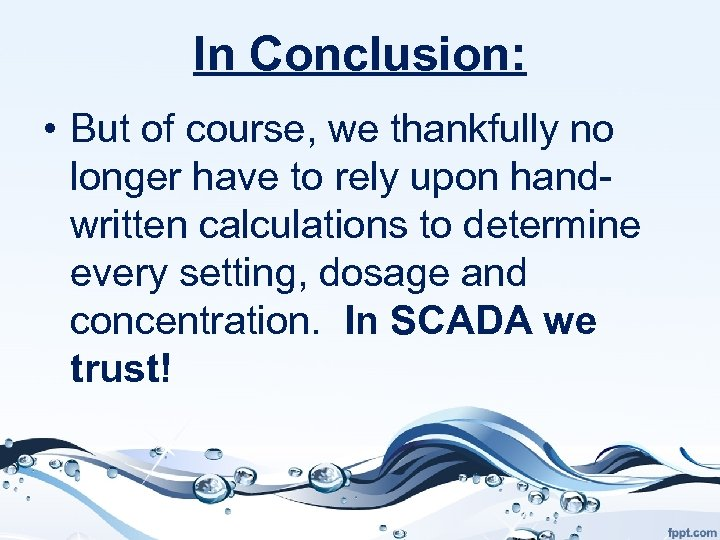In Conclusion: • But of course, we thankfully no longer have to rely upon handwritten calculations to determine every setting, dosage and concentration. In SCADA we trust!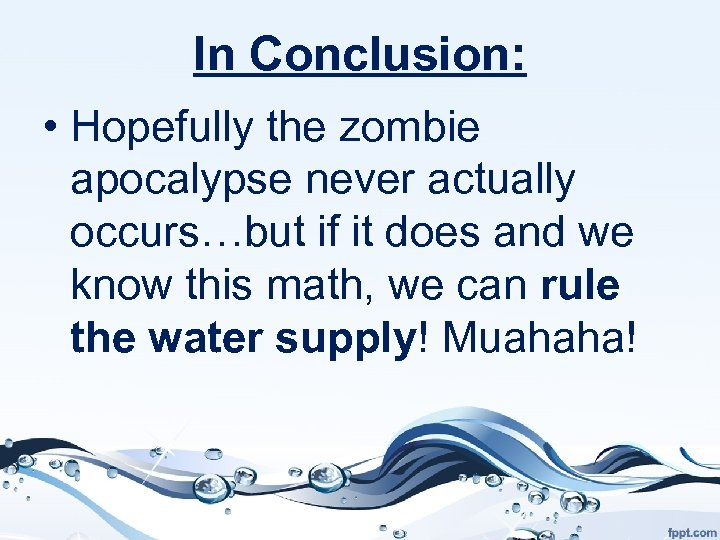In Conclusion: • Hopefully the zombie apocalypse never actually occurs…but if it does and we know this math, we can rule the water supply! Muahaha!Thank You! • Thank you so much for your time and attention! We are honored to be here! • Thanks to Bill Fowler, President of MWAA, for inviting us to present!Thank You! • A HUGE, special thank you to Kathy Bates- the Environmental Health and Water Quality Technology instructor at Milwaukee Area Technical College and our mentor!Are You an MATC Alumnus? • We are celebrating the Environmental Health and Water Quality Technology program’s 50 th anniversary on Tuesday, March 20 th 2018! • We would be honored if you joined us for our big party!Please Join Us! • Contact Kathy Bates • [email protected] edu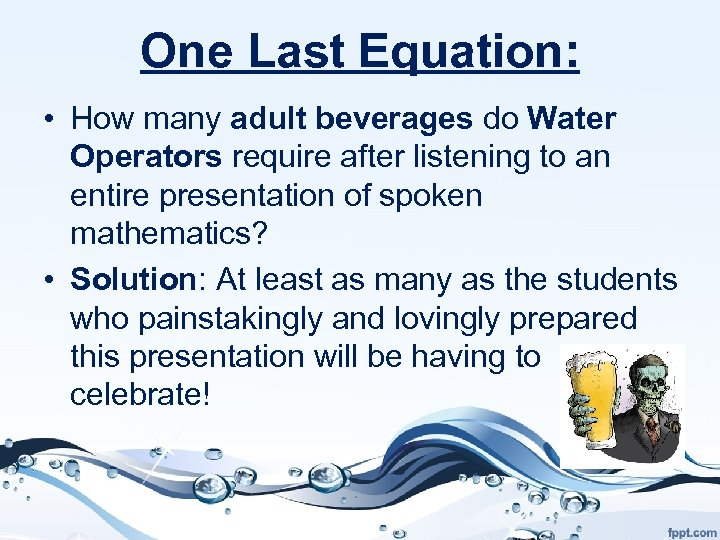One Last Equation: • How many adult beverages do Water Operators require after listening to an entire presentation of spoken mathematics? • Solution: At least as many as the students who painstakingly and lovingly prepared this presentation will be having to celebrate!